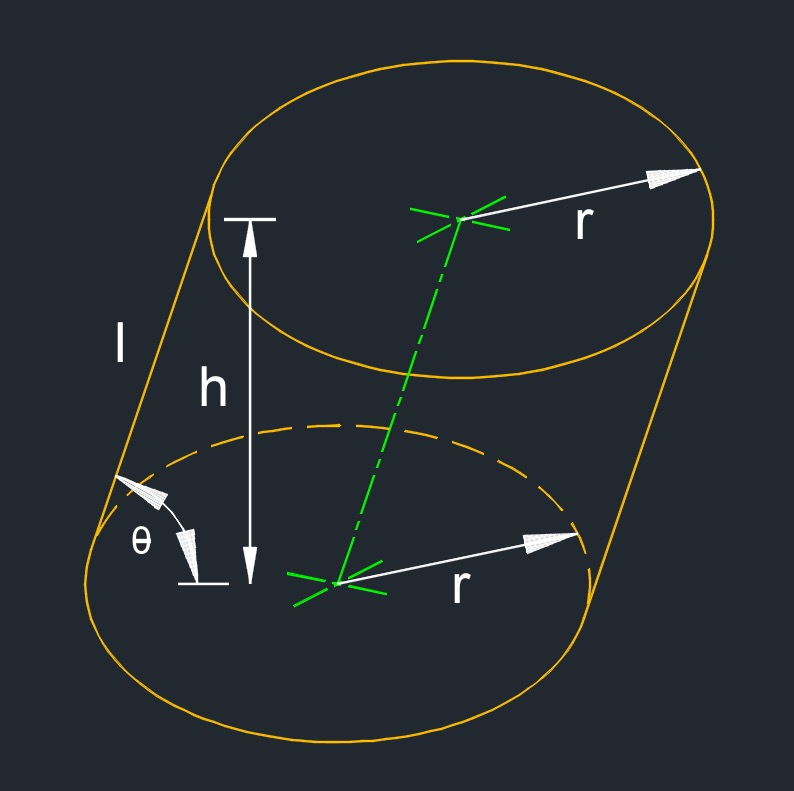# Oblique Cylinder

on . Posted in Solid Geometry

• Oblique cylinder (a three-dimensional figure) has both bases not alligned above each other and the center not at 90° to the other base center.
• 2 bases## Height of a Oblique Cylinder formula

$$\large{ h = l \; sin\;x }$$
Symbol English Metric
$$\large{ h }$$ = height $$\large{ in }$$ $$\large{ mm }$$
$$\large{ x }$$ = angle $$\large{ deg }$$ $$\large{ rad }$$
$$\large{ l }$$ = length $$\large{ in }$$ $$\large{ mm }$$## Lateral surface area of a Oblique Cylinder formula

$$\large{ A_l = 2\; \pi \; r \; l }$$
Symbol English Metric
$$\large{ A_l }$$ = lateral surface area (side) $$\large{ in^2 }$$ $$\large{ mm^2 }$$
$$\large{ l }$$ = length $$\large{ in }$$ $$\large{ mm }$$
$$\large{ \pi }$$ = Pi  $$\large{3.141 592 653 ...}$$
$$\large{ r }$$ = radius $$\large{ in }$$ $$\large{ mm }$$## Surface area of a Oblique Cylinder formula

$$\large{ A_s = l + 2 \; \pi \;r^2 }$$
Symbol English Metric
$$\large{ A_s }$$ = surface area (bottom, top, side) $$\large{ in^2 }$$ $$\large{ mm^2 }$$
$$\large{ l }$$ = length $$\large{ in }$$ $$\large{ mm }$$
$$\large{ \pi }$$ = Pi  $$\large{3.141 592 653 ...}$$
$$\large{ r }$$ = radius $$\large{ in }$$ $$\large{ mm }$$## Volume of a Oblique cylinder formula

$$\large{ V = \pi\; r^2\;h }$$
Symbol English Metric
$$\large{ V }$$ = volume $$\large{ in^3 }$$ $$\large{ mm^3 }$$
$$\large{ h }$$ = height $$\large{ in }$$ $$\large{ mm }$$
$$\large{ \pi }$$ = Pi  $$\large{3.141 592 653 ...}$$
$$\large{ r }$$ = radius $$\large{ in }$$ $$\large{ mm }$$